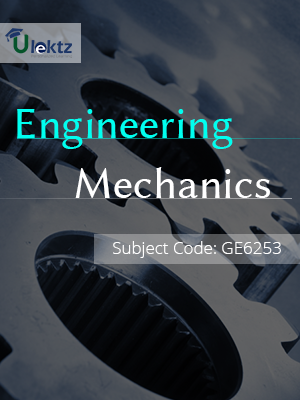•My WalletMy Order
•My Profile
•My Connections
•My Books
•My Videos
•My Tests
•My Calender
•My Messages
•My Shopping Cart
•My Orders
•Account Settings
•Help

# Book Details# Engineering Mechanics

 Course Code : GE6253 Author : uLektz University : Anna University, Tamil Nadu Regulation : 2013 Categories : General Engineering Format :ePUB3 (DRM Protected) Type : eBook

FREE

Description :Engineering Mechanics of GE6253 covers the latest syllabus prescribed by Anna University, Tamil Nadu for regulation 2013. Author: uLektz, Published by uLektz Learning Solutions Private Limited.

Note : No printed book. Only ebook. Access eBook using uLektz apps for Android, iOS and Windows Desktop PC.

##### Topics
###### UNIT I BASICS AND STATICS OF PARTICLES

1.1 Introduction

1.2 Units and Dimensions

1.3 Laws of Mechanics, Lami’s Theorem, Parallelogram and Triangular Law Of Forces

1.4 Vectorial representation of forces

1.5 Vector operations of forces, Additions, Subtraction, Dot Product, Cross Product

1.6 Coplanar Forces, Rectangular components, Equilibrium of a particle

1.7 Forces in space, Equilibrium of a particle in space

1.8 Equivalent systems of forces, Principle of transmissibility

###### UNIT II EQUILIBRIUM OF RIGID BODIES

2.1 Free body diagram,Types of supports, Action and reaction forces, Stable equilibrium

2.2 Moments and Couples, Moment of a force about a point and about an axis,Vectorial representation of moments and couples,Scalar components of a moment

2.3 Varignon‟s theorem, Single equivalent force, Equilibrium of Rigid bodies in two dimensions, Equilibrium of Rigid bodies in three dimensions

###### UNIT III PROPERTIES OF SURFACES AND SOLIDS

3.1 Centroids and centre of mass, Centroids of lines and areas, Rectangular,Circular, Triangular areas by integration, T section,I section,Angle section, Hollow section by using standard formula

3.2 Theorems of Pappus

3.3 Area moments of inertia of plane areas, Rectangular,Circular, Triangular areas by integration, T section, I section, Angle section, Hollow section by using standard formula

3.4 Parallel axis theorem and perpendicular axis theorem

3.5 Principal moments of inertia of plane areas, Principal axes of inertia

3.6 Mass moment of inertia, Mass moment of inertia for prismatic, cylindrical and spherical solids from first principle

3.7 Relation to area moments of inertia

###### UNIT IV DYNAMICS OF PARTICLES

4.1 Displacements,Velocity and acceleration, their relationship

4.2 Relative motion

4.3 Curvilinear motion

4.4 Newton’s law of motion

4.5 Work Energy Equation

4.6 Impulse and Momentum

4.7 Impact of elastic bodies

###### UNIT V FRICTION AND ELEMENTS OF RIGID BODY DYNAMICS

5.1 Friction force, Laws of sliding friction, Equilibrium analysis of simple systems with sliding friction,Wedge friction

5.2 Rolling resistance

5.3 Translation and Rotation of Rigid Bodies,Velocity and acceleration,General Plane motion of simple rigid bodies such as cylinder, disc/wheel and sphere

### Related Books